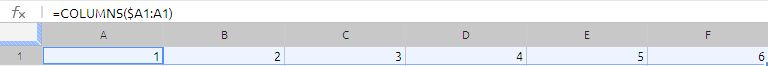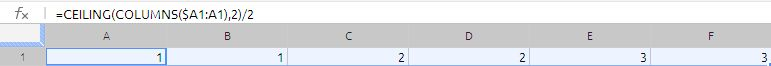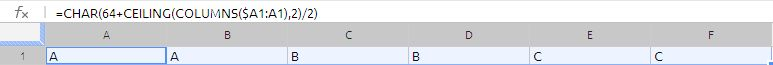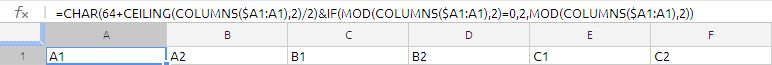## SERIES GENERATION IN GOOGLE DOCS

Question in the Forum:

i need to use data in a column and add two different text strings to each item. this then must be put into a row.
so if my data is (a,b,c,d,) i want the output in my row to be:    | a1 | a2 | b1 | b2 | c1 | c2 | d1 | d2 |.
i have used =TRANSPOSE(ARRAYFORMULA(A2:A5 & ” 1″)) to get | a1 | b1 | c1 | d1 |   but i can’t figure out how to also add the other string.

Suggested solution:

the solution suggested by me is as follows:

=CHAR(64+CEILING(COLUMNS(\$A1:A1),2)/2)&IF(MOD(COLUMNS(\$A1:A1),2)=0,2,MOD(COLUMNS(\$A1:A1),2))

now lets break down the formula

our first formula is

=COLUMNS(\$A1:A1)

if we drag this formula across the columns we will be as shown below imagesince \$A1 is relative reference with column locked this will act as expanding range and will generate the series of numbers from 1,2,3,4,5,6…. etc.

but our objective is to generate the series as 1,1,2,2,3,3… etc.  to convert the 1,2,3,4,5,6…etc into required series we have wrapped this in to CEILING function. now the formula is

=CEILING(COLUMNS(\$A1:A1),2)/2now we got the required series, to convert the required series into a alphabet we are using the CHAR function

The formula will be =CHAR(64+CEILING(COLUMNS(\$A1:A1),2)/2)Character 65 is A as per Ascii character set, we are using this to convert the number 1,1,2,2,3,3… etc into alphabits A,A,B,B,C,C……….etc

to get the number 1,2,1,2,1,2 we are using the if with MOD function,With use of MOD function we are converting the odd column numbers to 1 and even column numbers to 2.